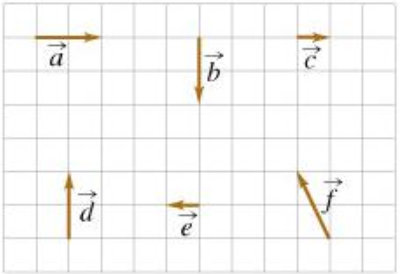# Problem: Six vectors (a through f) have the magnitudes and directions indicated in the figure.Part ARank the vector combinations on the basis of their magnitude.Rank from largest to smallest. To rank items as equivalent, overlap them.(F+C), (D), (A+E), (A+C), (A+D), (A+B)Part BRank the vector combinations on the basis of their angle, measured counterclockwise from the positive x axis. Vectors parallel to the positive x axis have an angle of 0 degrees. All angle measures fall between 0 and 360 degrees.Rank from largest to smallest. To rank items as equivalent, overlap them.(F+C) , (D), (A+E), (A+C), (A+D), (A+B)

###### FREE Expert Solution
89% (72 ratings)###### Problem Details

Six vectors (a through f) have the magnitudes and directions indicated in the figure.Part A

Rank the vector combinations on the basis of their magnitude.

Rank from largest to smallest. To rank items as equivalent, overlap them.

(F+C), (D), (A+E), (A+C), (A+D), (A+B)

Part B

Rank the vector combinations on the basis of their angle, measured counterclockwise from the positive x axis. Vectors parallel to the positive x axis have an angle of 0 degrees. All angle measures fall between 0 and 360 degrees.

Rank from largest to smallest. To rank items as equivalent, overlap them.

(F+C) , (D), (A+E), (A+C), (A+D), (A+B)